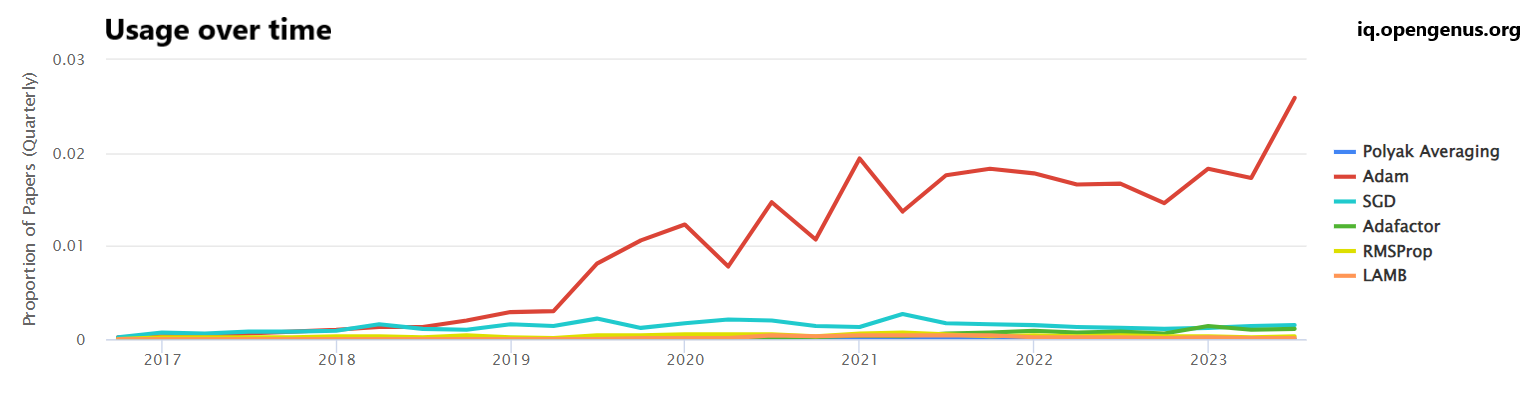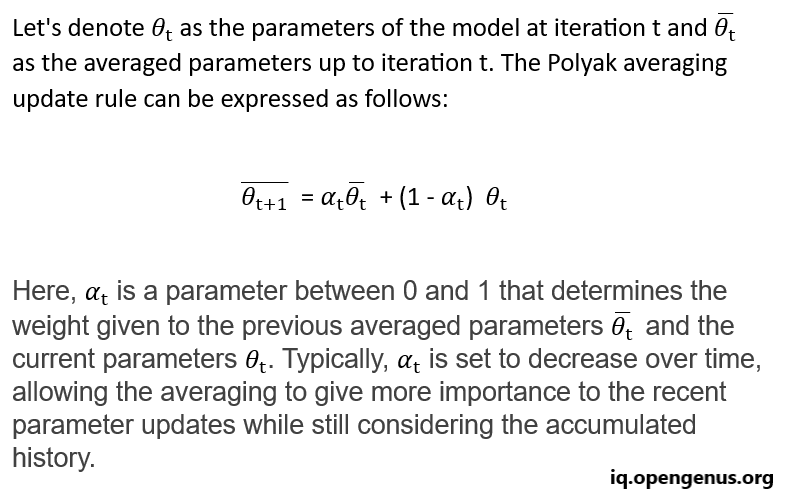×

Search anything:

# Polyak Averaging

#### Deep LearningGet this book -> Problems on Array: For Interviews and Competitive Programming

## Key Takeaways

• Polyak averaging, also known as Polyak-Ruppert averaging, is a technique used in machine learning to improve the performance and stability of models, especially in the context of deep learning.
• Polyak Averaging smoothens training by averaging model parameters, reducing sensitivity to noisy updates.
• Averaging parameters improves the model's ability to generalize, leading to better performance on unseen data.
• In reinforcement learning, it balances exploring new actions and exploiting existing knowledge, improving learning in dynamic environments.
• Helps stabilize parameter oscillations during training, ensuring more consistent updates and preventing erratic behavior.
• Averaging weights facilitates a more stable convergence of the model, reducing the risk of overshooting optimal solutions.

• Background
• What does Optimization Mean?
• Polyak Averaging Technique
• Applications of Polyak Averaging

Polyak averaging is named after its inventors Anatoli Juditsky and Arkadii Nemirovski (also known as Polyak and Ruppert) and is a method of model averaging that helps to produce a more robust and accurate final model by combining the information from different iterations of the model during the training process.

### Background:

In the training process of machine learning models, especially deep neural networks, the model parameters are iteratively updated to minimize a certain loss function. During this process, the model may oscillate around optimal parameters due to noise in the data or stochasticity in the optimization algorithm. These fluctuations can prevent the model from converging to the true optimal solution.

### What does Optimization Mean?

Optimization refers to the method of discovering the most effective solution to a problem. Within mathematics, optimization problems often revolve around determining the highest or lowest value of a function. A typical instance involves identifying the briefest route between two locations on a map. Optimization finds applications across diverse fields such as engineering, finance, and computer science.

### Polyak Averaging Technique:

Polyak averaging addresses this issue by maintaining a running average of the model parameters throughout the training process. Instead of using the parameters from the final iteration as the solution, Polyak averaging computes a weighted average of the model parameters obtained during all iterations. This averaging smoothens out the parameter updates and helps in reducing the impact of noise and oscillations, leading to a more stable and accurate final model.

Polyak Averaging provides numerous advantages to optimization algorithms. One crucial benefit is its ability to mitigate overfitting. Overfitting occurs when an algorithm becomes excessively intricate, fitting the noise in the data rather than the actual pattern. By computing the average of recent parameters, Polyak Averaging smooths the algorithm's trajectory, minimizing the risk of overfitting.

Additionally, Polyak Averaging accelerates the convergence of optimization algorithms. Convergence in optimization denotes finding the optimal solution. By averaging recent parameters, Polyak Averaging refines the search area, enabling algorithms to converge more swiftly and efficiently.

Here's a more in-depth explanation of how Polyak averaging works:

• Objective Function and Optimization Algorithm:
• Suppose you have an optimization problem, such as training a machine learning model. In this context, you have a loss function that you want to minimize, typically representing he difference between your model's predictions and the actual target values.
• You employ an optimization algorithm, like stochastic gradient descent (SGD), Adam, or RMSprop, to update the model's parameters iteratively.• Noisy or Fluctuating Objective Function:
• In real-world scenarios, the objective function may not be smooth and can exhibit fluctuations due to various factors like noisy data, batch sampling variability, or inherent randomness in the problem.
• Optimization algorithms can sometimes get stuck in local minima or exhibit oscillatory behavior when dealing with such non-smooth and fluctuating functions.
• Parameter Averaging:
• Instead of considering only the final set of parameters obtained after a fixed number of optimization iterations, Polyak averaging introduces the concept of maintaining two sets of parameters:
• Current Parameters: These are the parameters that the optimization algorithm actively updates during each iteration.
• Averaged Parameters: These are the parameters obtained by averaging the current parameters over multiple iterations.
• Mathematically:Example,
Let's say we have two weights from two neural networks: W1 from one network, and W2 is the corresponding weight from the other network. Polyak averaging averages these weights as follows:
``````   W_average = W1 * p + W2 * (1-p)
When p is 0.5, it's a evenly weighted average. If p is high, then W1 is weighted more heavily than W2, etc.
``````
• Final Output:
• After a certain number of iterations or when the optimization process converges, you use the averaged parameters as the final model.
• The averaged parameters are expected to be more stable and less susceptible to fluctuations or noise in the optimization process.
• By using the averaged parameters as the final model, you often achieve better generalization and a more reliable solution, especially when dealing with complex and noisy optimization landscapes.

• Improved Generalization: Averaging the parameters helps in finding a solution that generalizes better to unseen data. It reduces the risk of overfitting by producing a smoother and more stable model.
• Reduced Sensitivity to Hyperparameters: Polyak averaging can make the model less sensitive to the learning rate and other hyperparameters, as the averaging process mitigates the impact of abrupt parameter changes.
• Enhanced Robustness: By reducing the impact of noisy updates, Polyak averaging makes the training process more robust, especially in scenarios where the data is noisy or the optimization landscape is complex.

### Applications of Polyak Averaging

Polyak averaging is particularly useful in deep learning, where finding an optimal set of parameters is challenging due to the high dimensionality of the model. By providing a stable and accurate solution, Polyak averaging contributes significantly to the training of deep neural networks.#### Rishi Shivhare

Data Science Enthusiast | Manipal University Jaipur - 2024